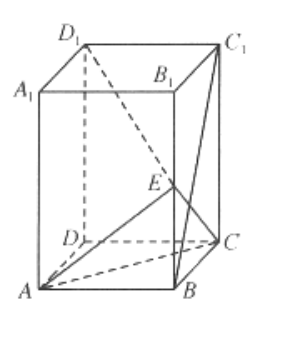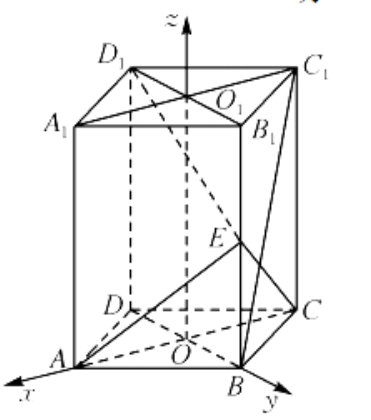(1)求证: $A C \perp D_1 E$;
(2) 若二面角 $A-E C-B$ 的余弦值为 $\frac{\sqrt{6}}{4}$, 求直线 $B C_1$ 与平面 $A E C$ 所成角的正 弦值.【答案】 (1) 证明: 连接 $B D, B_1 D_1$, 如图所示. 因为四边形 $A B C D$ 是菱形, 所以 $A C \perp B D$, 因为直四棱柱 $A B C D-A_1 B_1 C_1 D_1$, 所以 $B B_1 \perp$ 平面 $A B C D$, メ $A C \subset$ 平面 $A B C D$, 所以 $B B_1 \perp A C$, 义 $B D \cap B B_1=B, B D, B B_1 \subset$ 平面 $D B B_1 D_1$, 所以 $A C \perp$ 平面 $D B B_1 D_1$,

(2) 解: 记 $A C$ 与 $B D$ 的交点为 $O$, 连接 $A_1 C_1$ 交 $B_1 D_1$ 于点 $O_1$.